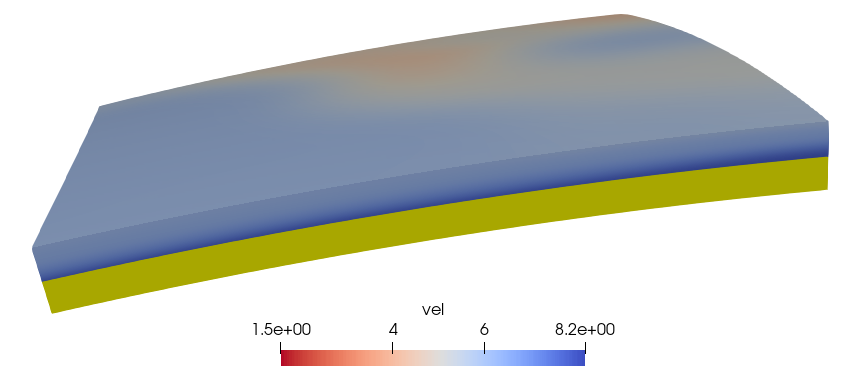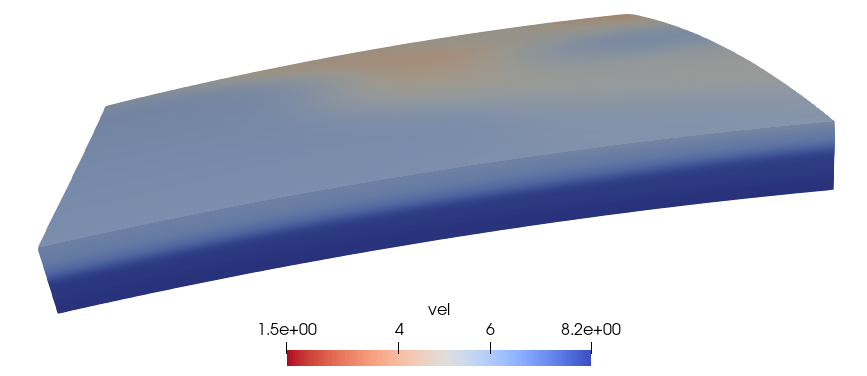## 1. 将原始模型线性插值到自定义网格

  1 2 3 4 5 6 7 8 9 10  import numpy as np from scipy.interpolate import griddata new_dep, new_lat, new_lon = np.meshgrid(dep, lat, lon, indexing='ij') grid_vp = griddata( points[:, 0:3], points[:, 3], (new_dep, new_lat, new_lon), method='linear' )## 2. 用临近点插值将NaN值点赋值

  1 2 3 4 5 6 7 8 9 10 11 12 13  index = np.where(~np.isnan(data)) values = data[index] points = np.array(index).T zidx = np.arange(data.shape) yidx = np.arange(data.shape) xidx = np.arange(data.shape) zz, xx, yy = np.meshgrid(zidx, yidx, xidx, indexing='ij') interpolated = griddata( points, values, (zz, xx, yy), method='nearest' ) result = interpolated.reshape(data.shape)